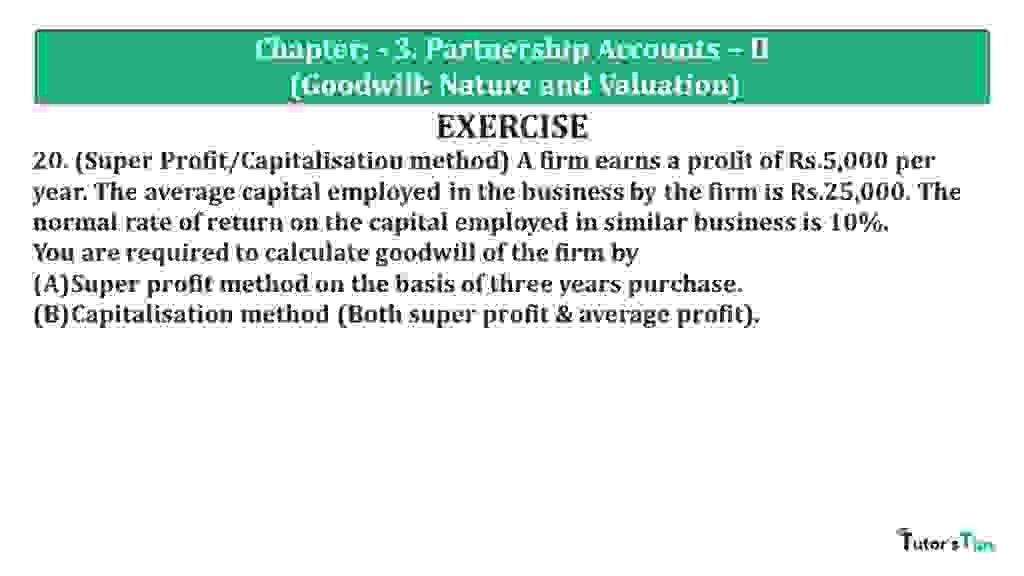# Question 20 Chapter 3 of +2 Part-1 – USHA Publication 12 Class Part – 1Question 20 Chapter 3 of +2-Part-1

20. (Super Profit/Capitalisation method) A firm earns a profit of Rs.5,000 per year. The average capital employed in the business by the firm is Rs.25,000. The normal rate of return on the capital employed in similar business is 10%.
You are required to calculate goodwill of the firm by
Super profit method on the basis of three years purchase.
Capitalisation method (Both super profit & average profit).

### The solution of Question 20 Chapter 3 of +2 Part-1: –

 Actual Profits p.a = Rs.5,000 Capital Employed = Rs. 25,000 Rate of return = 10%

 Normal Profit = Capital Employed X Normal Rate of Return 100 = 25,000 X 10 100 = 2,500

i) Super Normal Profit Method

 Super Profit = Actual Profit – Normal Profit = 5,000 – 2,500 = 2,500

 Number of years’ purchase = 3 Goodwill = Super Profit X Number of years’ purchase = 2,500 X 3 = 7,500

ii) Capitalisation Method:

a) Capitalisation of Super Profits Method:

 Goodwill = Super Profit X 100 Normal Rate of Return = 2,500 X 100 10 = 25,000

b) Capitalisation of Average Profits Method:

 Goodwill = Super Profit X 100 Normal Rate of Return = 5,000 X 100 10 = 50,000
 Super Profit = Capitalised value of the business – Average Capital Employed = 50,000 – 25,000 = 25,000